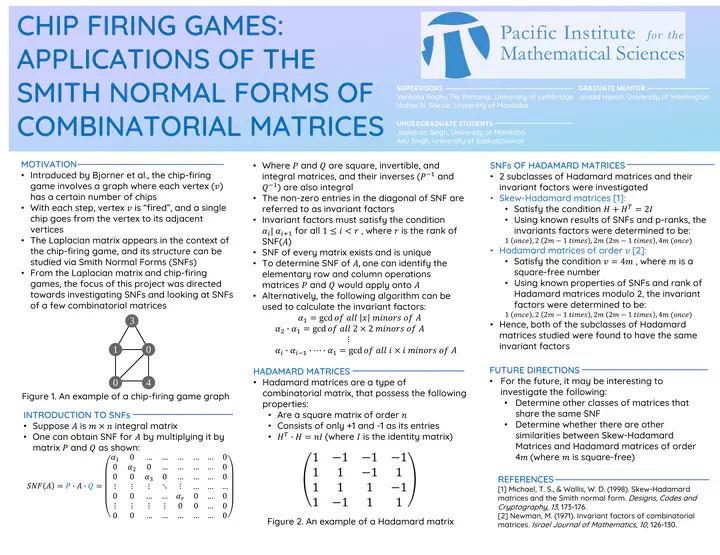# Chip firing games: applications of the Smith Normal Forms of Combinatorial matricesPoster for “Chip Firing Games: Applications of the Smith Normal Forms of Combinatorial Matrices”

• Junaid Hasan, University of Washington

• Jaskaran Singh, University of Manitoba
• Anu Singh, University of Saskatchewan

## Problem Statement

The Smith Normal Forms (SNF) of an incidence matrix is a powerful invariant that may help distinguish the underlying incidence structure. Of special interest is the SNF of the Laplacian matrix of a graph. This comes up in the context of the popular chip-firing game. This game was introduced by Bjorner et al. Fix a graph on n vertices and start by putting chips $a\left\{i\right\}$ at the $i$th vertex. So $a=(a\left\{1\right\}, … a\left\{i\right\}, … , a\left\{n\right\})$ is in $Z_\left\{+\right\}^{n}$, let $N$ be the sum of $a\left\{i\right\}$’s. Each step involves firing a vertex $v$, that is, one chip from $v$ goes to each of its adjacent vertices. A vertex $v$ can be fired if the number of chips currently held at $v$ is at least the degree of $v$. A position of size $N$ on $G$ is a distribution of $N$ chips to vertices of $G$. A legal game is any sequence of positions, such that each position is obtained from the previous one by firing at a vertex of $G$. Bjorner et al proved that given a connected graph, with an initial distribution of chips, either every legal game can be continued indefinitely, or terminated after the same number of steps and final position. Biggs introduced a variant of this game in which a vertex $q$ can have a negative number of chips, in fact, we need $q$ to always be in “debt”. A configuration on $G$ is a distribution of $s(j)$ chips at every vertex j≠q, and s(q)=-sum s(j) chips at q. Biggs proved that any starting configuration of a graph $G$ leads to a unique critical configuration, and the set of critical configurations has a natural group operation that is isomorphic to the critical group $K(G)$.

Potential research plan: Literature indicates that the class of Strongly regular graphs (SRG) has been amenable to the computation of critical groups. There have been many papers computing the critical groups of various classes of SRGs. Quite a few of these have been coauthored by undergraduate researchers. Participating in this program will be encouraged to pick a class of SRGs and perform computer experiments to conjecture the structure of critical group

For more details please see the full project proposal.

### Final Report

This VXML project was successfully completed by the participants listed above, as described in the following documents

### Details

• Expected team size: 3
• Student Experience Level: Intermediate: students who have an introduction to proofs
##### Prerequisites
• Linear Algebra
##### Skills
• Some techniques from ring theory and representation theory of groups, but students will be able to learn these concepts on the go.##### Venkata Raghu Tej Pantangi
###### Postdoctoral Fellow, University of Lethbridge